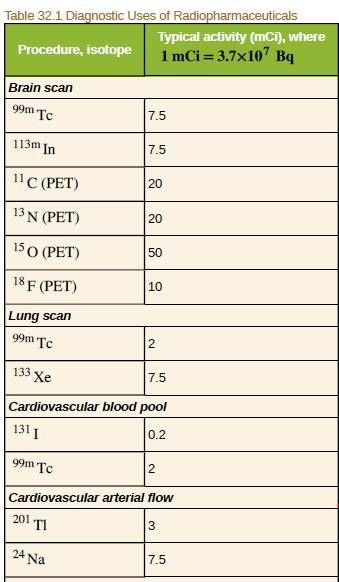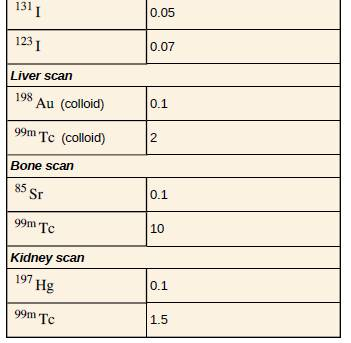Chapter 32, Problem 6PE

Chapter
Section
Textbook Problem

The activities of 131I and 123I used in thyroid scans are given in Table 32.1the 50 and 70 μCi, respectively. Find and compare the masses of 13lI and 231I in such scans, given their respective half−lives are 8.04 d and 13.2 h. The masses are so small than the radioiodine is usually mixed with stable iodine as a carrier to ensure normal chemistry and distribution in the body.To determine

The comparison between the masses of I131 and I123.

Explanation

Given info:

The activity of I131 is

A=0.05m·Ci

The half-life of I131 is

T1/2=8.04day=8.04×24×3600sec

The activity of I123 is

A=0.07m·Ci

The half-life of I123 is

T1/2=13.2h=13.2×3600sec

Formula used:

The mass can be found as

m=NNa×molarmass

Calculation:

Mass of I131

The activity

A=0.05m·Ci=0.05×3.70×107BqA=1.85×106Bq

Activity is defined as

A=Nln2T 1 2N=AT1/2ln2

Substituting the values

N=(1.85×106Bq)×8.04×24×3600secln2N=1.85×1012atoms

Substituting all values, the mass of mI131 is

mI131=1.85×1012atoms6.02×1023atoms×131gmI131=4.03×1010g

Mass of I123

The activity

A=0.07m·Ci=0

Still sussing out bartleby?

Check out a sample textbook solution.

See a sample solution

The Solution to Your Study Problems

Bartleby provides explanations to thousands of textbook problems written by our experts, many with advanced degrees!

Get Started

Find more solutions based on key concepts